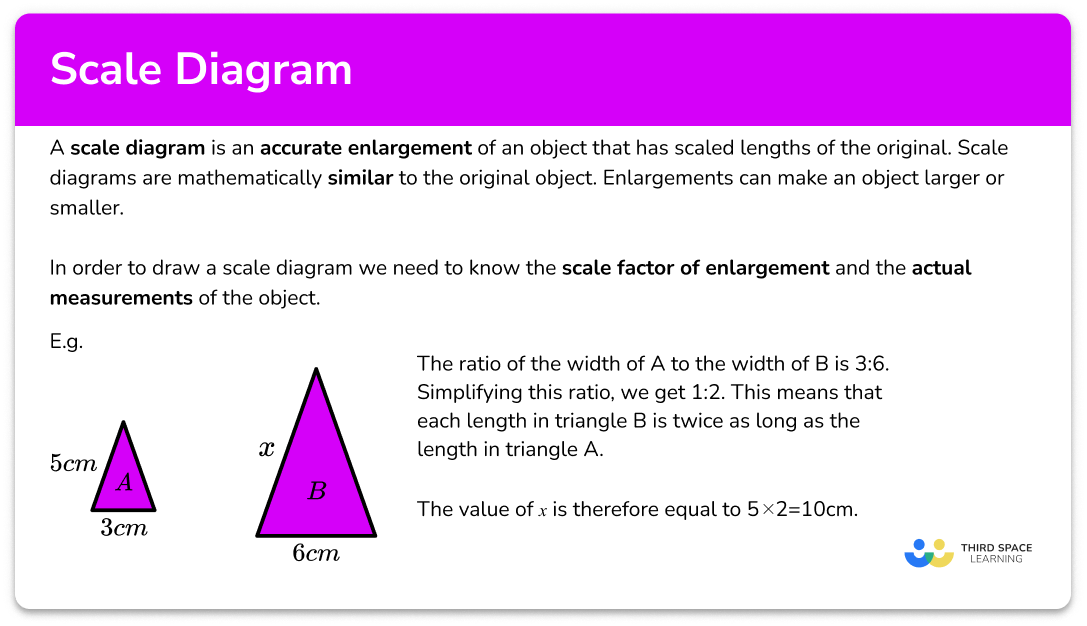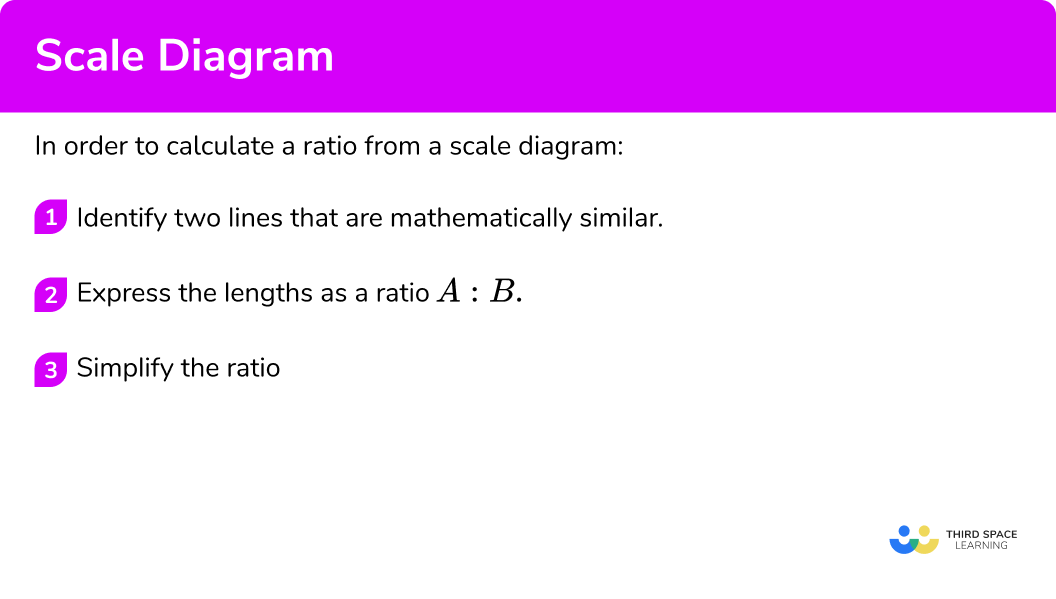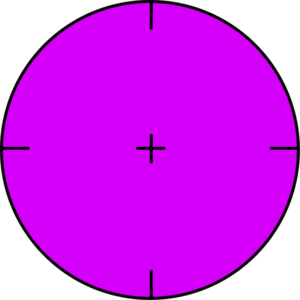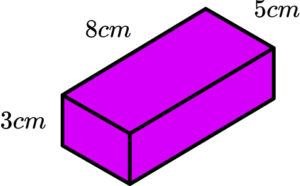# Scale Diagram

Here we will learn about a scale diagram, including similar shapes, using scale factors, and geometric problems.

There are also scale diagrams and drawings worksheets based on Edexcel, AQA and OCR exam questions, along with further guidance on where to go next if you’re still stuck.

## What is a scale diagram?

A scale diagram is an accurate enlargement of an object that has scaled lengths of the original. Scale diagrams are mathematically similar to the original object.

Enlargements can make an object larger or smaller.

In order to draw a scale diagram we need to know the scale factor of enlargement and the actual measurements of the object.

E.g.

Below are two similar triangles, \mathrm{A} and \mathrm{B}.

Triangle \mathrm{A} has a width of 3cm , and a slant height of 5cm.

Triangle \mathrm{B} has a width of 6cm and a slant height of x.

To find the value of x we need a scale factor (which we can express as a ratio).

Comparing two similar dimensions, the ratio of the width of \mathrm{A} to the width of \mathrm{B} is 3:6. Simplifying this ratio, we get 1:2. This means that each length in triangle \mathrm{B} is twice as long as the length in triangle \mathrm{A}.

The value of x is therefore equal to 5 \times 2=10cm.

### What is a scale diagram?## How to calculate a ratio from a scale diagram

In order to calculate a ratio from a scale diagram:

1. Identify two lines that are mathematically similar.
2. Express the lengths as a ratio \bf{\mathrm{A:B}} .
3. Simplify the ratio.

### Explain how to calculate a ratio from a scale diagram## Related lessons on scale

Scale diagram is part of our series of lessons to support revision on scale. You may find it helpful to start with the main scale lesson for a summary of what to expect, or use the step by step guides below for further detail on individual topics. Other lessons in this series include:

## Scale diagram examples

### Example 1: a straight line

A straight line on a road is measured as 5.7m. The diagram shows a scaled version of the line is represented below.

State the ratio of the diagram to the actual distance of the line.

1. Identify two lines that are mathematically similar.

Here we have the two lines with the lengths 5.7m and 15cm.

2Express the lengths as a ratio \bf{\mathrm{A:B}} .

The ratio is of the form \text{diagram : actual} so we have the ratio

15cm:5.7m

3Simplify the ratio.

Converting the right hand side of the ratio to be in centimetres we have

15cm:570cm

Since the units are the same we can rewrite the ratio without them

15:570

Writing the ratio in the simplest form we have:

1:38

### Example 2: 2D polygon

The scale diagram of a parallelogram is 12cm wide and has a vertical height of 10cm. The real life structure is 42cm wide. State the ratio of the scale diagram to the real life structure.

Identify two lines that are mathematically similar.

Express the lengths as a ratio \bf{\mathrm{A:B}} .

Simplify the ratio.

### Example 3: 3D object

The diagram of Cube \text{A} has a volume of 64cm^3 . Cube \text{A} has an actual volume of 27cm^{3}. State the ratio of the side lengths of the model to the actual size.

Identify two lines that are mathematically similar.

Express the lengths as a ratio \bf{\mathrm{A:B}} .

Simplify the ratio.

### Example 4: map

The distance between two points on a map is 8.6cm. The actual distance between the two points is 2.15km. Calculate the map scale in the form 1:n where both values are in centimetres.

Identify two lines that are mathematically similar.

Express the lengths as a ratio \bf{\mathrm{A:B}} .

Simplify the ratio.

### Example 5: floor plan

Below is the scale diagram of a floor plan of a bedroom. The length of the bed is 190cm. State the ratio of the floor plan to the actual distances in its simplest form.

Identify two lines that are mathematically similar.

Express the lengths as a ratio \bf{\mathrm{A:B}} .

Simplify the ratio.

### Example 6: scale model

A scale model of the planets in the solar system is given below. The minimum distance from Earth to Mars is 54.6 \times 10^{6}km from the surface of each planet. State the ratio in the form 1cm:n \ km of the scale model to the real distance.

Write n in standard form correct to 3 significant figures.

Identify two lines that are mathematically similar.

Express the lengths as a ratio \bf{\mathrm{A:B}} .

Simplify the ratio.

### Common misconceptions

• The order of the ratio is incorrect

Stating the ratio in the incorrect order will mean that the scale diagram is much larger / smaller than what is expected.

E.g. Let a ratio represent the scale diagram to the real life distance. The ratio 1:2 means that the real life distance is twice the length of the scale diagram.

The ratio 2:1 means that the real life distance is half of the scale diagram.

• Incorrect units in the solution

The units for the model can be mixed up with the units for the real life distance.

E.g.

If we were calculating the distance of 10cm on a map with the scale ratio of 1cm:5km, \ 10 \times 5=50cm is stated whereas the correct solution would be 10 \times 5=50km.

• Converting units

Sometimes the units in a question need to be converted so that they are all the same, so it is important to be confident in converting between different metric units.

E.g. The map scale is given as 1:25000 which means that 1cm on the map is equivalent to 25000cm in real life.

If the answer is asked to be written in kilometres, the real life value in centimetres must be divided by 100 \ 000 to get the same measurement in kilometres.

### Practice scale diagram questions

1. The height of a fence post is 2m. Below is a scale diagram of the same fence post. State the ratio of the scale diagram to the actual fence post in the form 1:n. Give your answer in its simplest form.1cm:2m2:110cm:20cm1:20First we need to write down the ratio with the given information.

10cm:2m

Then we can convert so that they have the same units.

10cm:200cm

Finally, we can simplify the ratio.

10:200=1:20

2. A circle has a diameter of 16.4cm. The diagram below shows a scale model of the same circle. State the ratio of the scale diagram to the actual circle.1cm:2.05m1:2.052:11m:20.5cm\begin{aligned} &8cm:16.4cm \\\\ &1cm:2.05cm=1:2.05 \end{aligned}

3. The volume of a cube shaped tank is 125m^{3}. The cross section of the scale drawing has an area of 36cm^{2}. State the ratio of the side lengths of the scale diagram to the actual width of the tank.

50cm:6cm5:66:5006:5A cube with a volume of 125m^3 has a side length of \sqrt{125}=5m

A cube with a cross sectional area of 36cm^2 has a side length of \sqrt{36}=6cm

6cm:5m=6cm:500cm

4. The distance between two places on a map is 5.3cm. The actual distance between the two places is 2.65km. Calculate the map scale in the form 1:n where both units are in centimetres

1:250001:0.51:21:5000\begin{aligned} &2.65km=265000 cm \\\\ &265000 \div 5.3=50000 \\\\ &1:50000 \end{aligned}

5. The length of a desk in an office is twice the length of the bookcase. If the desk is 2.2m long in real life, and the bookcase is 5.5cm long on the diagram, state the ratio of real life to the scale diagram.

5:120:12:540cm:1m\begin{aligned} &5.5 \times 2=11cm \quad \text{(desk length on diagram)} \\\\ &2.2m:11cm \\\\ &220cm:11cm \\\\ &220:11 \\\\ &20:1 \end{aligned}

6. A miniature toy dinosaur is being put into production. The average length of a diplodocus is 25m. The height of the back legs of a diplodocus are 18\% of its length. The back legs of the scale model are 1.5cm tall. What is the simplified ratio of the model to the actual dinosaur?

5:11:3002:5001.5cm:25m\begin{aligned} &25 \times 0.18=4.5m \\\\ &1.5cm:4.5m \\\\ &1.5cm:450cm \\\\ &1.5:450 \\\\ &1:300 \end{aligned}

### Scale diagram GCSE questions

1. The length of a path is measured at 26.2 metres. A scale drawing of the same path has a length of 14 \ cm. State the ratio of the diagram to the actual path in the form 1:n.

(2 marks)

14cm:26.2 m =14:2620

(1)

1:187

(1)

2. A camera screen has a height of 7cm and a width of 5cm.

A photo frame is 19cm wide.

(a) What is the scale ratio of the camera screen to the photo frame?

(b) Calculate the height of the photo frame.

(4 marks)

(a)

5:19

(1)

1:3.8

(1)

(b)

7 \times 3.8

(1)

26.6cm

(1)

3. A box of chocolates has the following dimensions:A scale model is created. The ratio of the real object to the model is 1:0.2. Calculate the volume of the model chocolate box. State the units in your answer.

(3 marks)

(3 \times 0.2) \times (8 \times 0.2) \times (5 \times 0.2)

(1)

0.96

(1)

cm^3

(1)

## Learning checklist

You have now learned how to:

• Use scale factors, scale diagrams and maps
• Solve problems involving similar shapes where the scale factor is known or can be found

## Still stuck?

Prepare your KS4 students for maths GCSEs success with Third Space Learning. Weekly online one to one GCSE maths revision lessons delivered by expert maths tutors.

Find out more about our GCSE maths tuition programme.